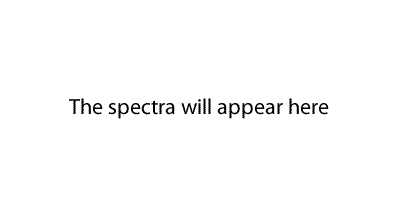Exercises should be attempted when you have covered NMR, IR and mass spectra. The table of IR absorption data may help identify some bonds. Click on the blue writing to reveal the spectra. An organic compound was analysed and found to contain the following percentage composition by mass. 36.36%O, 9.10%H, 54.54%C. A 4.4 gram sample of the pure compound was found to contain 3.01 X10 22 molecules. 1) Calculate the empirical formula of the compound 2) Find the molecular formula of the compound. 3) Identify the molecule by drawing its molecular structure given its IR and NMR spectra.   solution 4) Butanoic acid has the same molecular formula as the unknown compound. Will the NMR spectrum be enough to eliminate butanoic acid? Explain 5) Draw the NMR spectrum of butanoic acid (CH3CH2CH2COOH) solutionAn organic compound was analysed and found to contain the following percentage composition by mass. 43.24%O, 8.11%H, 48.65%C. A 7.4 gram sample of the pure compound was found to contain 6.02 X10 22 molecules. 1) Calculate the empirical formula of the compound 2) Find the molecular formula of the compound. 3) Identify the molecule by drawing its molecular structure given its IR and NMR spectra. solution 4) Methyl ethanoate has a similar molecular formula as our unknown compound. Draw the NMR spectrum of methyl ethanoate. Indicate the TMS and the relative positions of the different hydrogens, taking into account nuclear shielding. solutionAn organic compound was analysed and found to contain the following percentage composition by mass. 28.32%O, 6.19%H, 53.10%C, 12.39%N. A sample containing 2.00 mol of the pure substance weighs 226.0 grams 1) Calculate the empirical formula of the compound 2) Find the molecular formula of the compound. 3) Identify the molecule by drawing its molecular structure given its IR and NMR spectra.   solutionAn organic compound was analysed and found to contain the following percentage composition by mass. 36.36%O, 9.10%H, 54.54%C. 1) Calculate the empirical formula of the compound 2) With reference to the mass spectrum find the molecular formula of the compound 3) Identify the molecule by drawing its molecular structure given its mass, NMR and IR spectra. solutionContinue